# Basic Geometric constructions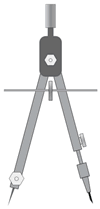With just a compass and a straightedge (or virtual versions of them), you’ll be amazed by how many geometric shapes you can construct perfectly.This is the “pure” form of geometric construction: no numbers involved.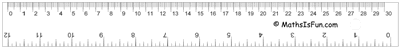## Basic Geometric constructions

 Mostly used things were these two ⇓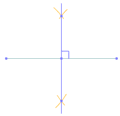Line Bisector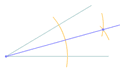Angle Bisector And it is useful to know how to do 30°, 45° and 60° angles. We can use the angle bisector method (above) to create other angles, such as 15°, etc: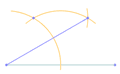30 Degree Angle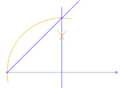45 Degree Angle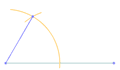60 Degree Angle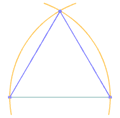Equilateral Triangle Points and Lines: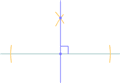Perpendicular to a Point on a Line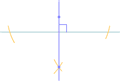Perpendicular to a Point NOT on a Line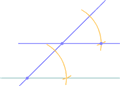Parallel Line through a Point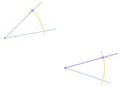Same (Congruent) Angle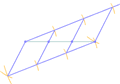Cut a line into N segments Circles: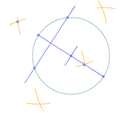Center of a Circle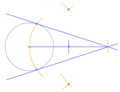Point to Tangents on a Circle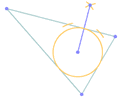Inscribe a Circle in a Triangle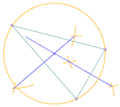Circumscribe a Circle on a Triangle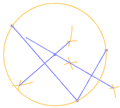Circle touching 3 Points And for the “Master Class”, a Pentagon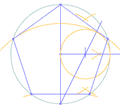Pentagon